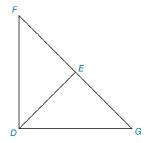Chapter 3.2, Problem 27E### Elementary Geometry for College St...

6th Edition
Daniel C. Alexander + 1 other
ISBN: 9781285195698

#### Solutions

Chapter
Section### Elementary Geometry for College St...

6th Edition
Daniel C. Alexander + 1 other
ISBN: 9781285195698
Textbook Problem
32 views

# In Exercise 27 to 29, prove the indicated relationship.Given: D F ¯ ≅ D G ¯ and F E ¯ ≅ E G ¯ Prove: D E → bisects ∠ F D G

To determine

To prove:

The given statement.

Explanation

Given:

The following figure shows the given diagram.

Given that,

DF¯DG¯ and FE¯EG¯

Approach:

By definition of SSS, triangles will be congruent if all three sides in one triangle is congruent to all three sides of second triangle.

The completed proof is shown in the following table.

 PROOF Statements Reasons 1. DF¯≅DG¯ and FE¯≅EG¯ 1. Given 2. DE¯≅DE¯ 2. Identity 3. ΔFDE≅ΔGDE 3. SSS 4. ∠FDE≅∠GDE 4. CPCTC 5. DE→ bisects ∠FDG 5

### Still sussing out bartleby?

Check out a sample textbook solution.

See a sample solution

#### The Solution to Your Study Problems

Bartleby provides explanations to thousands of textbook problems written by our experts, many with advanced degrees!

Get Started

#### Multiply: 6.121.32

Elementary Technical Mathematics

#### For f(x) = 5 + g(x), f(x) = _____. a) 5g(x) b) 5 + g(x) c) 0 g(x) d) g(x)

Study Guide for Stewart's Single Variable Calculus: Early Transcendentals, 8th

#### A normal vector to the plane 2x + 3y + 6z =–20 is:

Study Guide for Stewart's Multivariable Calculus, 8th